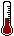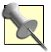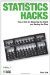# Hack 24. Produce PercentilesA simple but powerful way of understanding test performance is through the use of percentile ranks. Here's how to take a raw score with little explanatory value and transform it into something much more informative and useful.

In school, teachers (or counselors, or whoever reported standardized test results) might have reported results to you without ever telling you your score. Instead, you probably saw a number that looked like a percentage and was described as telling you how you (or your child) compared to others who took the test. This type of score is called a percentile rank.

If you have been shown a percentile rank that represents your test performance, it won't be useful unless you know what it means. On the flip side, if you have to explain someone's test performance and you show the test taker a raw score only, you aren't really being very helpful. Being able to build or interpret percentile ranks is a useful skill for both sides of the testing game.

Norm-referenced scoring [Hack #26] is an approach to making test scores more informative by comparing scores to each other. The norm-referenced score you see most often in the real world is the percentile rank. The percentile rank is defined as "the percentage of scores in a distribution that are less than a given score of interest." For example, if you get 15 items correct out of 20 on a quiz and half the class got fewer correct than you, your percentile rank is 50.

#### Producing and Reporting Percentile Ranks

If you are a classroom teacher, human resources manager, or anyone who has to report test results to others, being able to report a percentile rank instead of a raw score will help test takers understand how well they performed and also help decision makers understand the consequences of setting various standards of performance.

Producing percentiles begins with organizing all your test scores. For a small data set, it is fairly simple to build a frequency table, which answers all sorts of questions in addition to providing percentile ranks. Here is a sample distribution for 30 scores on a classroom test (arranged from lowest to highest) in which 100 points was the highest possible score:

59, 65, 72, 75, 75, 75, 80, 83, 83, 85, 85, 85, 85, 85, 85, 86, 86, 86, 86, 88, 88, 88, 90, 90, 90, 90, 90, 92, 94, 97
##### Compute frequencies and percentages

For efficiency's sake, this data can be displayed and the frequency of each score can be computed, as shown in Table 3-1.

##### Table Cumulative frequency for a classroom test
ScoreFrequencyCumulative frequencyPercentageCumulative percentage
59113.33 percent3.33 percent
65123.33 percent6.67 percent
72133.33 percent10.00 percent
753610.00 percent20.00 percent
80173.33 percent23.33 percent
83296.67 percent30.00 percent
8561520.00 percent50.00 percent
8641913.33 percent63.33 percent
8832210.00 percent73.33 percent
9052716.67 percent90.00 percent
921283.33 percent93.33 percent
941293.33 percent96.67 percent
971303.33 percent100.00 percent

Table 3-1 shows each score that someone actually got, how many people got that score, the total number of people getting a given score or lower, the percentage of all people getting each score, and the total percentage of people getting a given score or lower. The cumulative columns always report the total number of people (or scores) in the distribution (30 in our example) and the total percentage of people (always 100 percent).

##### Determine percentile ranks

To determine the percentile rank for any score in the distribution, use the "Cumulative percentage" column. Find the score of interest and look at the cumulative percentage in the row just above that score's row. For instance, for a score of 94, the percentile rank is 93.33 or about the 93rd percentile. For a score of 86, the percentile rank is 50.If you review a dozen statistics or measurement textbooks, you'll find that there are actually two different and competing definitions for a percentile rank. I prefer "the percentage of scores in a distribution that are less than a given score of interest," but some books give "the percentage of scores in a distribution that are equal to or less than a given score of interest." Both definitions are reasonable and percentile ranks can be calculated either way using a frequency table. Under the first use of the term, there can be no 100th percentile. Under the second, there can be no 0th percentile. Pick the definition you prefer and go with it, but always share your definition along with your results.

#### Interpreting the Percentile Rank

Imagine that you are sitting down with your guidance counselor and have been told that your percentile rank is 93. So, what does this mean? Well, the most direct interpretation is that 93 percent of all people who took the test scored less than you did. It is also correct to say that 7 percent of people scored equal to you or higher. We can also think of percentile ranks as saying how far the score is from normal. The mean percentile rank is usually around the 50th percentile and will be exactly that if scores are normally distributed, as they (ahem) normally are. So, we could also say that the 93rd percentile is pretty far above average.

Don't make the mistake that many otherwise savvy stat-hackers sometimes make. Earlier in this hack, we used an example of a test score in which you got 15 items correct out of 20 on a quiz and half the class got fewer correct than you. Your percentile rank in that example was 50. Notice that in that example, your percent correct is 75 percent (15/20), but your percentile rank is 50. Don't confuse the two! Knowing your percentile rank does not tell you how many questions you got right.

#### Where It Doesn't Work

Remember that a percentile rank is useful only when you're looking for a norm-referenced interpretation. If you want to know whether you have mastered a key set of skills, it does not help to know what percentage of people have mastered more or less of those skills. To know where you are compared to some set of standards, not compared to other people, you want a criterion-referenced score [Hack #26]. A percent correct type of score is more meaningful for you in this case than a percentile rank.

• If you assume that your scores are normally distributed, or at least drawn from a population that is normally distributed, you can just convert any standardized score directly to the percentile rank, using information about the areas under the normal curve [Hack #25].Statistics Hacks: Tips & Tools for Measuring the World and Beating the Odds
ISBN: 0596101643
EAN: 2147483647
Year: 2004
Pages: 114
Authors: Bruce Frey

Similar book on Amazon